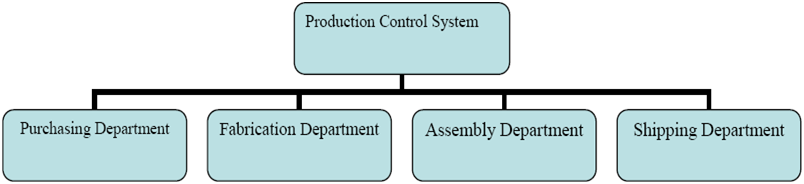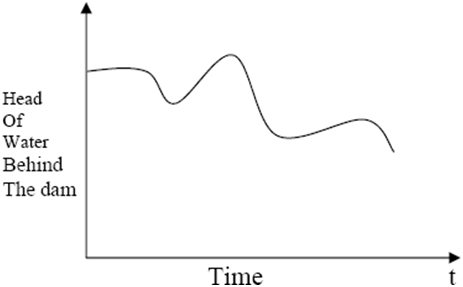### System And System Environment

• System:
A system is defined as an aggregation or assemblage of objects that are linked in some regular interaction or interdependence to achieve some goal.Example: Production System
There are several distinct objects in the preceding system, each with interesting properties. In addition, certain interactions occur in the system, causing changes in the system.

• System Environment:
The system environment is defined as the external components that interact with the system and cause necessary changes. It is necessary to decide on the boundary between the system and its environment when modelling systems. This decision may be influenced by the study's purpose.

• Endogenous System:
The term "endogenous" refers to actions and events that occur within a system.
For example:  withdrawing cash from a bank.

• Exogenous System:
Exogenous refers to actions and occurrences in the environment that have an impact on the system.
For example: Customers' arrival.

• Closed System:
A system that has no exogenous action or event is said to be closed.
For example: Water in an insulated flask.

• Open system:
An open system is one in which there is external activity and event.
For example:Bank system.

Components of a System:

• Entity:
An entity is a system object of interest.

Departments, orders, parts, and products are examples of entities in the factory system.

• Attribute:
An attribute denotes an entity's property.
For example, the quantity of each order, the type of part, or the number of machines in a Department are all attributes of a factory system.

• Activity:
An activity is any process that causes changes in a system.
For example, consider the department's manufacturing process.

• State of the System:
The state of a system is defined as the collection of variables required to describe a system at any time in relation to the study's objective. In other words, the state of the system is a description of all the entities, attributes, and activities as they currently exist.

• Event:
An event is defined as a single occurrence that has the potential to change the state of the system.

Discrete and Continuous Systems:

• Continuous Systems:
Continuous systems are those in which the changes are primarily smooth.
For example:Head of a water behind a dam.

​​​​​​​​​​​​​​Model of a system:

• A model is a representation of a system created for the goal of studying it. Only those parts of the system that affect the problem under inquiry should be considered. These elements are represented in a model, which is a system simplification by definition.

Types of Model:
The various types models are

1. Mathematical or Physical Model

2. Static Model

3. Dynamic Model

4. Deterministic Model

5. Stochastic Model

6. Discrete Model and Continuous Model

• Mathematical or Physical Model:
A system is represented using symbolic notation and mathematical equations.

• Static Model:
Monte-Carlo simulation is a representation of a system at a specific point in time.

• Dynamic Model:
Represents the evolution of systems across time.
Example: Simulation of a bank

• ​​​​​​​Deterministic Model:
There are no random variables present. They have a set of known inputs that will produce a set of unique outputs.
Example: Patients arrive at the dentist on time for their planned appointment.

page no:10

• Stochastic Model:
Inputs include one or more random variables. Random inputs produce random results.

• Discrete and Continuous Model:
Used in a similar fashion. Both discrete and continuous simulation models can be combined. The decision is based on the system's characteristics as well as the study's goal.

Example: Simulation of a bank involves random interarrival and service times.